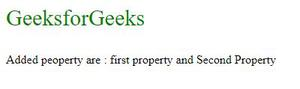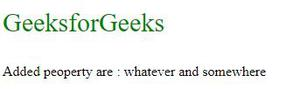# How to add a property to a JavaScript object using a variable as the name?

We can create a JavaScript object with an object literal:

Example :

` var obj = { firstname : "Romy", lastname : "kumari", age : 20}`

The name: values pairs in JavaScript objects are called properties. We can add the property to JavaScript object using a variable as the name by using dot notation or bracket notation.

Below example illustrate explain two different approaches:

Example 1: In this example, we will be using dot notation.

## HTML

 ` ` `<``html``> ` `    ``<``body``> ` `        ``<``p` `style``=``"font-size: 30px; color: green;"` `;> ` `          ``GeeksforGeeks ` `        `` ` ` `  ` `  `        ``<``p` `id``=``"add"``> ` ` `  ` `  `        ``<``script``> ` `            ``// Variable a and b ` `            ``var a = "string1"; ` `            ``var b = "string2"; ` `            ``var object1 = { firstname: "Romy",  ` `                            ``lastname: "Kumari" }; ` ` `  `            ``// Property using variable name ` `            ``object1.a = "first property"; ` `            ``object1.b = "Second Property"; ` ` `  `            ``document.getElementById("add").innerHTML =  ` `              ``"Added peoperty are : " + object1.a +  ` `              ``" and " + object1.b; ` `        `` ` `    `` ` ``

Output:Example 2: We can use the bracket operator if we want to add the property with the value stored in the variable. The obj.a is not same as obj[a]

## HTML

 ` ` `<``html``> ` `    ``<``body``> ` `        ``<``p` `style``=``"font-size: 30px; color: green;"``;> ` `          ``GeeksforGeeks ` `        `` ` ` `  ` `  `        ``<``p` `id``=``"add"``> ` ` `  ` `  `        ``<``script``> ` `            ``var myObj = new Object(); ` ` `  `            ``var a = "string1"; ` `            ``var b = "string2"; ` `            ``// This can be accesses with ` `            ``// the value stored in b. ` `            ``myObj.b = "somewhere"; ` `            ``// This can be accessed with the  ` `            ``// value stored in a that is string1 ` `            ``myObj[a] = "whatever";  ` ` `  `            ``document.getElementById("add").innerHTML =  ` `              ``"Added peoperty are : " + myObj.string1 + ` `              ``" and " + myObj.b; ` `        `` ` `    `` ` ``

Output:My Personal Notes arrow_drop_upCheck out this Author's contributed articles.

If you like GeeksforGeeks and would like to contribute, you can also write an article using contribute.geeksforgeeks.org or mail your article to contribute@geeksforgeeks.org. See your article appearing on the GeeksforGeeks main page and help other Geeks.

Please Improve this article if you find anything incorrect by clicking on the "Improve Article" button below.